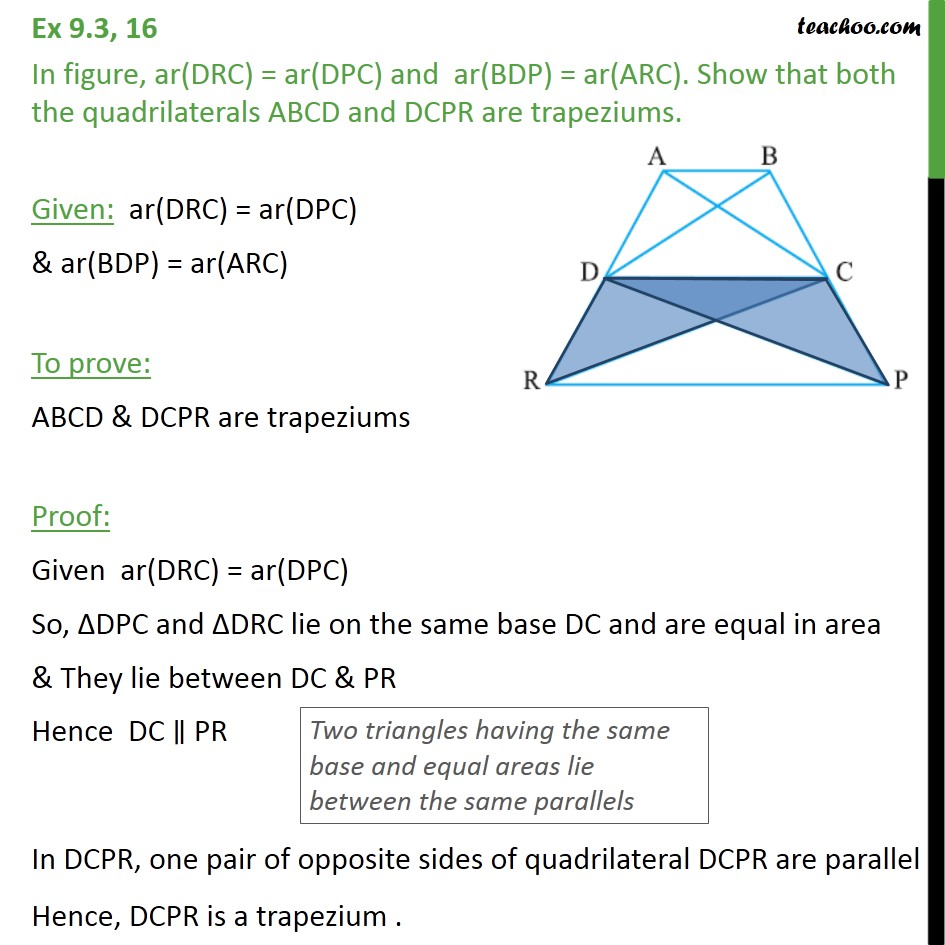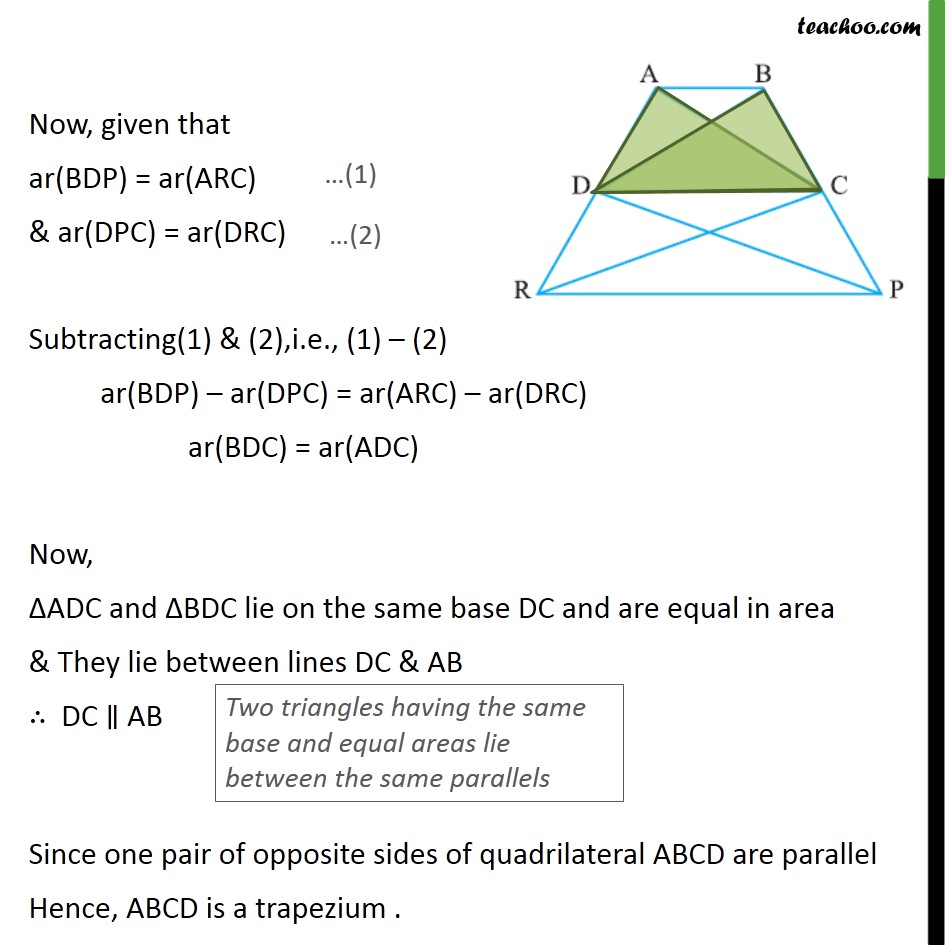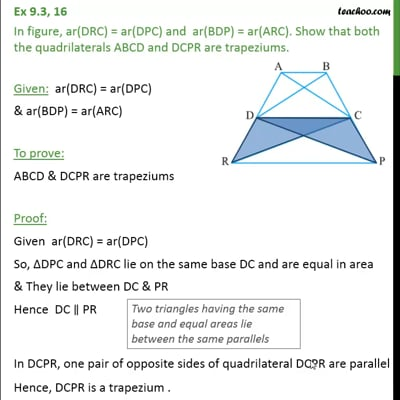Ex 9.3

Chapter 9 Class 9 - Areas of Parallelograms and Triangles
Serial order wiseThis video is only available for Teachoo black users

Introducing your new favourite teacher - Teachoo Black, at only ₹83 per month

### Transcript

Ex 9.3, 16 In figure, ar(DRC) = ar(DPC) and ar(BDP) = ar(ARC). Show that both the quadrilaterals ABCD and DCPR are trapeziums. Given: ar(DRC) = ar(DPC) & ar(BDP) = ar(ARC) To prove: ABCD & DCPR are trapeziums Proof: Given ar(DRC) = ar(DPC) So, DPC and DRC lie on the same base DC and are equal in area & They lie between DC & PR Hence DC PR In DCPR, one pair of opposite sides of quadrilateral DCPR are parallel Hence, DCPR is a trapezium . Now, given that ar(BDP) = ar(ARC) & ar(DPC) = ar(DRC) Subtracting(1) & (2),i.e., (1) (2) ar(BDP) ar(DPC) = ar(ARC) ar(DRC) ar(BDC) = ar(ADC) Now, ADC and BDC lie on the same base DC and are equal in area & They lie between lines DC & AB DC AB Since one pair of opposite sides of quadrilateral ABCD are parallel Hence, ABCD is a trapezium .